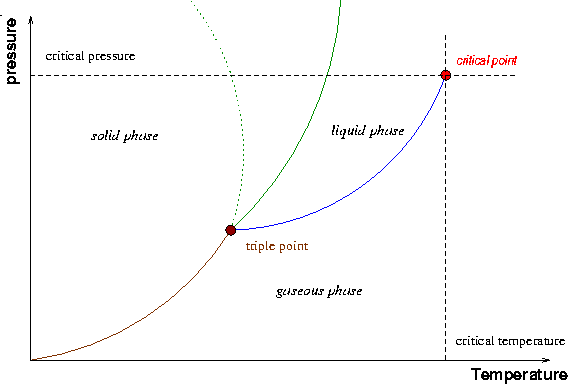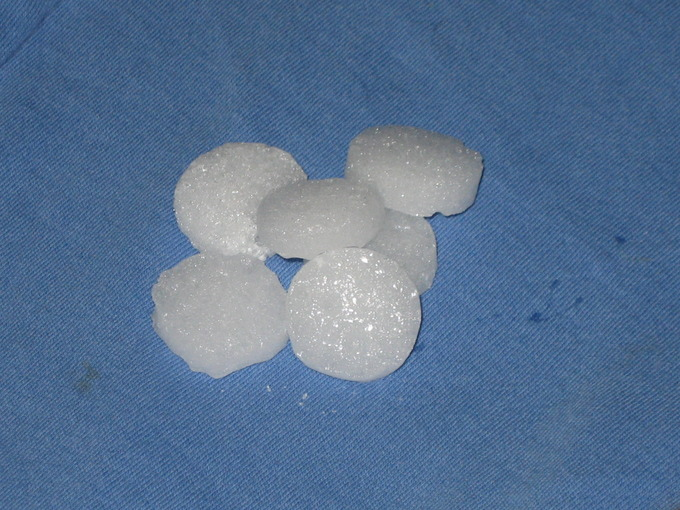## Major Features of a Phase Diagram

Phase diagrams are useful because they allow us to understand in what state matter exists under certain conditions.

### Learning Objectives

Describe the major features of a phase diagram.

### Key Takeaways

#### Key Points

• The major features of a phase diagram are phase boundaries and the triple point.
• Phase diagrams demonstrate the effects of changes in pressure and temperature on the state of matter.
• At phase boundaries, two phases of matter coexist (which two depends on the phase transition taking place).
• The triple point is the point on the phase diagram at which three distinct phases of matter coexist in equilibrium.

#### Key Terms

• Triple point: The unique temperature and pressure at which the solid, liquid, and gas phases of a substance are all in equilibrium with each other.
• phase boundary: The line in a phase diagram that indicates the conditions under which two (transitioning) states of matter exist at equilibrium.

A phase diagram is a graph which shows under what conditions of temperature and pressure distinct phases of matter occur. The simplest phase diagrams are of pure substances. These diagrams plot pressure on the y-axis and temperature on the x-axis.

Although phases are conceptually simple, they are difficult to define precisely. The phase of a system is generally defined as the region in the parameter space of the system’s thermodynamic variables (for immediate purposes, in the pressure-temperature parameter space) in which the system’s free energy is analytic (meaning it can be calculated exactly from known parameters of the system).

The major features of a phase diagram are phase boundaries and the triple point.

• Phase boundaries, or lines of equilibrium, are boundaries that indicate the conditions under which two phases of matter can coexist at equilibrium.
• The triple point is the point on the phase diagram where the lines of equilibrium intersect — the point at which all three distinct phases of matter ( solid, liquid, gas) coexist.Phase Diagram: In this phase diagram, which is typical of most substances, the solid lines represent the phase boundaries. The green line marks the freezing point (or transition from liquid to solid), the blue line marks the boiling point (or transition from liquid to gas), and the red line shows the conditions under which a solid can be converted directly to a gas (and vice-versa). The dotted green line is meant to replace the solid green line in the corresponding phase diagram of water. It illustrates water’s anomalous behavior.

The phase diagram for water is useful for learning how to analyze these diagrams. Along the blue phase boundary, water exists as both a vapor and a liquid. Along the dotted green phase boundary, we see the anomalous behavior of water: it exists as a solid at low-enough temperatures and high-enough pressures. At the triple point, water in the solid, liquid, and gaseous states coexist.

## Interpreting Phase Diagrams

Phase diagrams illustrate the effects selected variables of a system have on the state of matter.

### Learning Objectives

Interpret a phase diagram and explain its purpose

### Key Takeaways

#### Key Points

• Phase diagrams can be used to understand the range of thermodynamic variables over which a pure sample of matter exists as a particular state/phase.
• Phase diagrams are divided into three single phase regions that cover the pressure – temperature space over which the matter being evaluated exists: liquid, gaseous, and solid states.
• Phase diagrams can be used to understand under which conditions a pure sample of matter exists in two or three state equilibrium, by examining the phase boundaries and the triple point.

#### Key Terms

• critical point: The temperature and pressure at which the vapour density of the gas and liquid phases of a fluid are equal, at which point there is no difference between gas and liquid.
• supercritical fluid: A substance at a temperature and pressure above its own thermodynamic critical point that can diffuse through solids like a gas and dissolve materials like a liquid.

Phase diagrams illustrate the effects selected variables of a system have on the state of matter. Phase diagrams are divided into three single phase regions that cover the pressure-temperature space over which the matter being evaluated exists: liquid, gaseous, and solid states. The lines that separate these single phase regions are known as phase boundaries. Along the phase boundaries, the matter being evaluated exists simultaneously in equilibrium between the two states that border the phase boundary.

By focusing attention on distinct single phase regions, phase diagrams help us to understand the range over which a particular pure sample of matter exists as a particular phase. By examining the phase boundaries and the triple point, researchers can use phase diagrams to understand under which conditions a pure sample of matter exists in two or three state equilibrium. Phase diagrams can also be used to explain the behavior of a pure sample of matter at the critical point.A Typical Phase Diagram: A typical phase diagram illustrating the major components of a phase diagram as well as the critical point. The dotted green line refers to the solid-liquid phase boundary for water.

General observations from the diagram reveal that certain conditions of temperature and pressure favor certain phases of matter. Typically:

• Low temperature/high pressure conditions favor the solid state.
• Moderate temperature/moderate pressure conditions favor the liquid state.
• High temperature/low pressure conditions favor the gaseous state.

The critical point, which occurs at critical pressure (Pcr) and critical temperature (Tcr), is a feature that indicates the point in thermodynamic parameter space at which the liquid and gaseous states of the substance being evaluated are indistinguishable. At this point and beyond it, the substance being evaluated exists as a “supercritical fluid”. At temperatures above the critical temperature, the kinetic energy of the molecules is high enough so that even at high pressures the sample cannot condense into the liquid phase.

When evaluating the phase diagram, it is worth noting that the solid-liquid phase boundary in the phase diagram of most substances has a positive slope. This is due to the solid phase having a higher density than the liquid, so that increasing the pressure increases the melting temperature. However, the solid-liquid phase boundary for water is anomalous, in that it has a negative slope. This reflects the fact that ice is lower in density than liquid water (a well-known fact: ice floats), unlike most other substances which typically have denser solid phases.Mothballs – Phase Transitions Applied: The thermodynamic properties of mothballs, made of 1,4-Dichlorobenzene, are used to repel insects. 1,4- Dichlorobenzene sublimates (transitions from solid to gas) at room temperature. The gas released is toxic to insects.

With a knowledge of the major components of phase diagrams and the features of phase plots, a phase diagram can be used to understand how altering thermodynamic parameters influences the states/phases of matter a sample of a substance is in.